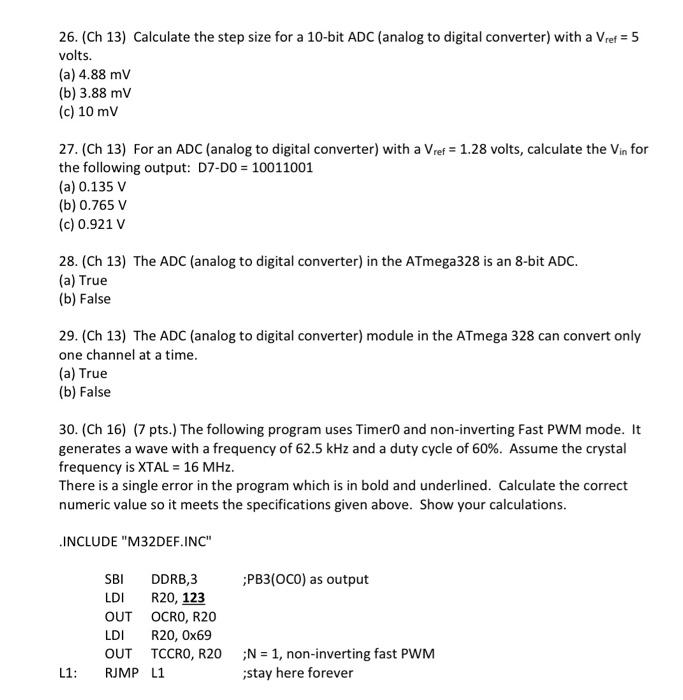# (Solved): all 26. (Ch 13) Calculate the step size for a 10-bit ADC (analog to digital converter) with a Vref ...

all26. (Ch 13) Calculate the step size for a 10-bit (analog to digital converter) with a volts. (a) (b) (c) 27. (Ch 13) For an (analog to digital converter) with a volts, calculate the for the following output: D7-D0 (a) (b) (c) 28. (Ch 13) The ADC (analog to digital converter) in the ATmega328 is an 8-bit ADC. (a) True (b) False 29. (Ch 13) The ADC (analog to digital converter) module in the ATmega 328 can convert only one channel at a time. (a) True (b) False 30. (Ch 16) (7 pts.) The following program uses Timer0 and non-inverting Fast PWM mode. It generates a wave with a frequency of and a duty cycle of . Assume the crystal frequency is . There is a single error in the program which is in bold and underlined. Calculate the correct numeric value so it meets the specifications given above. Show your calculations.

We have an Answer from Expert

26.To calculate the step size for a 10-bit ADC with a V_ref of 5 volts, we divide the voltage range by the number of possible output levels.
Voltage range = V_ref = 5 volts
Number of possible output levels = 2^10 = 1024

Step size = Voltage range / Number of possible output levels
= 5 volts / 1024
? 4.88 mV

Therefore, the correct answer is (a) 4.88 mV.